## Vector Outer Product

Vector outer product is denoted by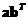or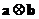. Vector outer product is also called vector matrix product because the result of the vector multiplication is a matrix. It can only be performed for two vectors of any size, not necessarily the same size.

Algebraically, the vector outer product is a multiplication of a column vector by a row vectorto obtain a matrix provided by the formula below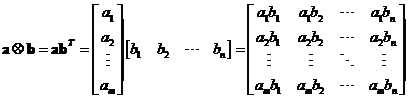Example
Suppose we have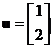and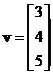, the vector outer products are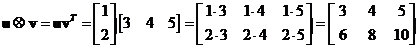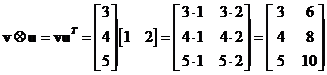Try to experiment with the interactive program below. Given two vectors, not necessarily at the same dimension, the program will produce the matrix outer product. If you click "Random Example" button, the program will generate random input vectors in the right format.

vector x vector y

## Properties

Some important properties of vector outer product are

• Vector outer product is a not commutative operation. If you reverse the order you will get different result and even different size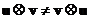.
• If matrix is the results of a vector product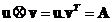then multiplication of that matrix with the second factor produces a vector of the first factor scaled by the square norm of the second factor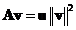.